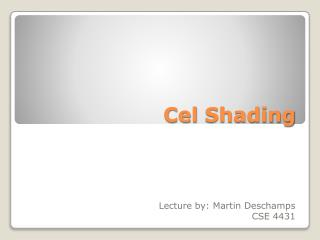DownloadDownload PresentationDownload Presentation- - - - - - - - - - - - - - - - - - - - - - - - - - - E N D - - - - - - - - - - - - - - - - - - - - - - - - - - -
##### Presentation Transcript

1. Cel Shading Lecture by: Martin Deschamps CSE 4431

2. Essentially, it is a type of non-photorealistic rendering designed to make computer graphics appear to be hand-drawn. Cel-shading is often used to mimic the simplistic style and feel of a hand drawn comic book or cartoon. • The name originates from the clear sheets of acetate, called cels, which are painted on for use in traditional 2D animation. • The style is more typically seen in cartoons, but a recent trend has cel-shading used more and more in recent video games and graphic applications. • Can also be referred to as Toon-shading What is cel shading?

3. Use of non-photorealistic shading means system processing is kept to a minimum • OpenGL 2.0 and GLSL 1.2 System Requirements

4. Seen here is a representation of Tintin’s Space exploring suit pictured in both cell shading and your everyday normal 3d shader. Normal vsCelShading Comparison

5. Examples

6. The cosine of the face’s normal is used to determine the brightness level. Closest to direction of light gets brighter tones.cos(lightDir,normal) = dot(lightDir , normal) / ( |lightDir| * |normal| ) • If both light dir and normal are normalized, above is simplified to :cos(lightDir,normal) = dot(lightDir, normal) • If given a OpenGL variable for the lights position instead of the uniform lightDir, we normalize it before using it in the dot product that forms intensity values. So our vertex shaderwhould look like this: • Vec3 lightDir = normalize(vec3(gl_LightSource.position));intensity = dot(lightDir, gl_Normal);gl_Position = ftransform(); The Basics

7. Because the intensity is set up as a varying variable used by both vertex and fragment shaders, we must first write it in the vertex shader so that the fragment shader can use it. The fragment shader would then follow a similar form to this: • vec4 color;if ( intensity > 0.95 ) color = vec4( 1.0, 0.5, 0.5, 1.0 );else if ( intensity > 0.5 ) color = vec4( 0.6, 0.3, 0.3, 1.0 );else if ( intensity > 0.25 ) color = vec4( 0.4, 0.2, 0.2, 1.0 );else color = vec4( 0.2, 0.1, 0.1, 1.0 );gl_FragColor = color; The Basics pt2

8. uniform vec3 lightDir; varying vec3 intensity;  void main(){ float intensity; vec4 color; intensity = dot(lightDir, gl_Normal); if (intensity > 0.95) color = vec4(1.0,0.5,0.5,1.0); else if (intensity > 0.5) color = vec4(0.6,0.3,0.3,1.0); else if (intensity > 0.25) color = vec4(0.4,0.2,0.2,1.0); else color = vec4(0.2,0.1,0.1,1.0); gl_FragColor = color; } In this case we compute the face normals in the vertex shader before passing the values to the fragment shader. It works but results are not optimal. uniform vec3 lightDir; varying float intensity; void main(){ intensity = dot(lightDir, gl_Normal); gl_Position = ftransform(); } Per Vertex Normalization

9. uniform vec3 lightDir;varying vec3 normal;void main(){ float intensity; vec4 color; intensity = dot(lightDir,normalize(normal)); if (intensity > 0.95) color = vec4(1.0,0.5,0.5,1.0); else if (intensity > 0.5) color = vec4(0.6,0.3,0.3,1.0); else if (intensity > 0.25) color = vec4(0.4,0.2,0.2,1.0); else color = vec4(0.2,0.1,0.1,1.0); gl_FragColor = color;} The difference between this new fragment shader and the one above is that we are now computing the normals in the fragment shader and not the vertex shader. We therefore get proper direction and unit length instead of just proper direction. Our vertex shader in this case essentially becomes two lines:varying vec3 normal; void main() { normal = gl_Normal; gl_Position = ftransform(); } Per Fragment Normalization

10. varying vec3 normal; void main(){ float intensity; vec4 color; vec3 n = normalize(normal); intensity = dot(vec3(gl_LightSource.position, n); if (intensity > 0.95) color = vec4(1.0,0.5,0.5,1.0); else if (intensity > 0.5) color = vec4(0.6,0.3,0.3,1.0); else if (intensity > 0.25) color = vec4(0.4,0.2,0.2,1.0); else color = vec4(0.2,0.1,0.1,1.0); gl_FragColor = color;} A way to simplify things a bit and use built in OpenGL Light attributes to save having to specify Light details in lightDir, we would modify our fragment shader to utilize said predetermined parameters as indicated below.varying vec3 normal; void main() { normal = gl_NormalMatrix * gl_Normal; gl_Position = ftransform(); } Using Light Sourcewith Parameters

11. uniform vec3 LightDir; uniform sampler2D Texture0; varying vec3 normal; vec4 CelShading (vec4 color){ float intensity = dot (LightDir, normalize (normal));  float factor = 1.0; if (intensity <0.5) factor = 0.5;  color *= vec4 (factor, factor, factor, 1.0);  return color; } void main (void){  vec4 color = Texture2D (Texture0, vec2(gl_TexCoord )); //additional effects can be added here color = CelShading( color ); gl_FragColor = color; } The benefit of this method allows for a segregation of the shading steps and opens opportunity for additional effects. The vertex shader also changes slightly to take the texture information into consideration. varying vec3 normal; void main(){ //Front color gl_FrontColor = gl_Color; //determine the normal of the vertex normal = gl_NormalMatrix * gl_Normal; //Texture coordinates gl_TexCoord = gl_MultiTexCoord0; //The position of the vertex gl_Position = ftransform(); } Use of Texture Coordinates in Shader

12. vec4 CelShading (vec4 color) { float intensity = dot(LightDir, normalize (normal));  float factor = 0.5;  if (Intensity> 0.95) factor = 1.0; else if (Intensity> 0.5) factor = 0.7; else if (Intensity> 0.25) factor = 0.4; else if (Intensity < 0.1 ) factor = 0.0;//rough outline approximation color *= vec4 (factor, factor, factor, 1.0);  return color; } Gradient Increments Simplified